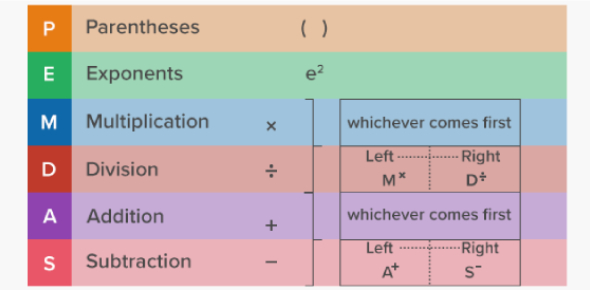# Order Of Operations Quiz: MCQ Test!

10 Questions | Total Attempts: 658Settings.

• 1.
5 − (9 − 3) + 6
• A.

5

• B.

10

• C.

6

• D.

-5

• E.

4

• 2.
8 ÷ 1 × 6) ÷ 6 − 4 =
• A.

5

• B.

4

• C.

1

• D.

8

• 3.
(9 ÷ 3 + 7 − 9 + 7)=
• A.

18

• B.

5

• C.

8

• D.

27

• 4.
2 + 7 × (7 × 9) ÷ 3=
• A.

149

• B.

89

• C.

179

• D.

49

• 5.
(4 × 6) − 8 =
• A.

18

• B.

18

• C.

22

• D.

16

• 6.
(1 − 8) × 3 =
• A.

-21

• B.

21

• C.

22

• D.

-22

• 7.
(4 ÷ 1 + 4) =
• A.

7

• B.

9

• C.

10

• D.

8

• 8.
(3 × 7 − 4 − 1 + 8) =
• A.

22

• B.

24

• C.

21

• D.

34

• 9.
(9 ÷ 1) − 7 =
• A.

1

• B.

3

• C.

2

• D.

4

• 10.
(5 × 9) − (5 − 7 × 6) =
• A.

12

• B.

82

• C.

92

• D.

72

Related TopicsBack to top